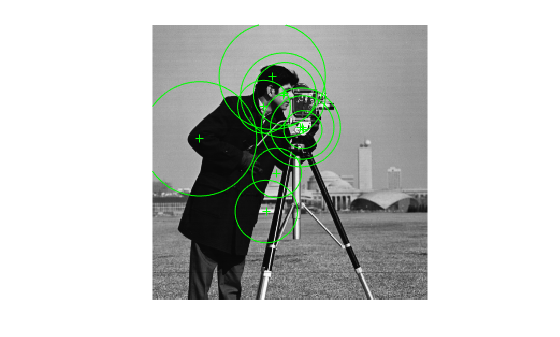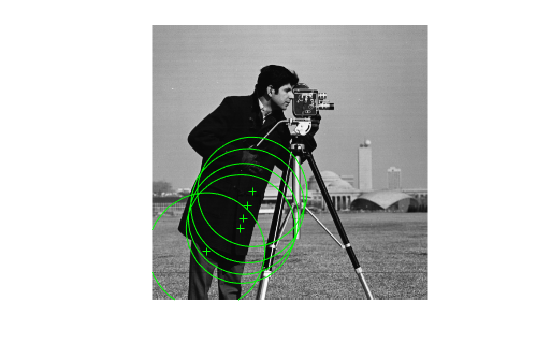Main Content

# KAZEPoints

Object for storing KAZE interest points

## Description

This object provides the ability to pass data between the `detectKAZEPoints` and extractFeatures functions. You can also use this object to manipulate and plot the data returned by these functions.Use the object to fill points interactively, where you might want to mix a non-KAZE interest oint detector with a KAZE descriptor.

## Creation

### Syntax

``points = KAZEPoints(location)``
``points = KAZEPoints(location,Name,Value)``

### Description

example

````points = KAZEPoints(location)` constructs a `KAZEPoints` object from an M-by-2 array [x y] of location coordinates. The scalar KAZEPoints object contains many points. Therefore `numel``(KAZEPoints)` always returns `1`. This value can be different than the result of `length(KAZEPoints)`, which returns the true number of points contained in the object.```

example

````points = KAZEPoints(location,Name,Value)` sets properties using one or more name-value pairs. Enclose each property name in quotes. For example, ```points = KAZEPoints('Metric',0.0)``````

### Input Arguments

expand all

Location of points, specified as an M-by-2 array of [x y] coordinates.

## Properties

expand all

Scale, specified as a scalar. The scale sets the size at which the interest points are detected.

Strength of response for the detected points, specified as a numeric value. The KAZE algorithm uses a determinant of an approximated Hessian.

Orientation of the detected feature, specified as an angle in radians. The angle is measured from the x-axis with the origin set by the `location` input. The `extractFeatures` function sets this property. Do not set it manually.

## Object Functions

 `isempty` Determine if points object is empty `length` Number of stored points `plot` Plot points `selectStrongest` Select points with strongest metrics `size` Return the size of a points object `selectUniform` Select uniformly distributed subset of feature points

## Examples

collapse all

Detect KAZE features and display 10 strongest points.

Read an image.

`I = imread('cameraman.tif');`

Detect KAZE features in the image.

`points = detectKAZEFeatures(I);`

Select the 10 strongest points.

`strongest = selectStrongest(points,10);`

Display the selected points.

``` imshow(I); hold on;```

Display the location and scale. The size of the circles displayed relate to the scale.

```plot(strongest); hold on;```Display the [x y] coordinates for the strongest points in the MATLAB Command Window.

`strongest.Location`
```ans = 10x2 single matrix 138.5041 95.8063 139.9253 95.8802 111.8975 48.2950 106.4036 174.1800 44.3964 106.4899 122.0368 65.9064 116.2702 138.2877 123.6542 64.7193 104.2719 76.5821 140.6228 97.9271 ```

Detect KAZE features and display set the specific KAZE points you want to plot.

Read an image.

`I = imread('cameraman.tif');`

Detect KAZE features in the image.

`points = detectKAZEFeatures(I);`

Select and display the last 5 points detected.

```imshow(I); hold on; plot(points(end-4:end)); hold off;```## Extended Capabilities

Introduced in R2017b

Download ebook